# Algebra II : Adding and Subtracting Fractions

## Example Questions

1 2 7 8 9 10 11 12 13 15 Next →

### Example Question #141 : Adding And Subtracting Fractions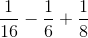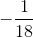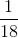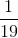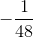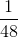Explanation:

In order to add and subtract fractions we must first find a common denominator before proceeding. The simplest way to begin is by taking the two fractions together, which have the smallest denominators, finding the common denominator between them, and adding/subtracting them, then finding the common denominator between this new fraction and the remaining fractions you have to add and subtract until the expression given is fully simplified.

Let's first look at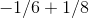:

Bothandcan be multiplied by a number to produce, which is the lowest common shared factor, thereforeis the lowest common denominator.would also work and allow you to obtain the same result, you'd just have to simplify it an extra step in the end to get the same result. When doing this it best to just think about what each of the numbers produces  through multiplication and see if you can quickly find a common factor, otherwise you can just multiply the two numbers together and simplify the expression later if you are short on time.

Since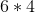, you would multiply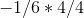to create a common denominator of, therefore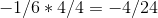Since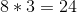you would multiply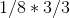to create a common denominator ofwhich gives you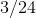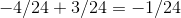Next we can look at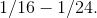Let's once again look for the lowest common denominator. Although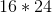is a common denominator there is a simpler solution, as bothandcan be multiplied by a number to produce.would be multiplied byto produceso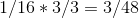is multiplied byto produce.

so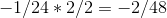Finally you can simplify the expressions to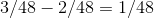which is your final answer.

### Example Question #171 : Fractions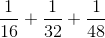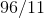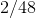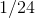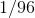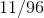Explanation:

In order to add and subtract fractions we must first find a common denominator before proceeding. The simplest way to begin is by taking the two fractions together, which have the smallest denominators, finding the common denominator between them, and adding/subtracting them, then finding the common denominator between this new fraction and the remaining fractions you have to add and subtract until the expression given is fully simplified.

Let's first look at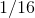and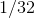:

Bothandcan be multiplied by a number to produceand the smallest common shared factor is, therefore it is the lowest common denominator.would also work but would require an additional simplification step to obtain the same answer. When looking for lowest common denominators it's best to just think about what multiplication each of the numbers produces and see if you can quickly find a common factor, otherwise you can just multiply the two numbers together and simplify the expression later if you are short on time.can be multiplied byto produce, therefore you would multiplyby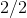, which gives you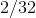.is multiplied byto produce, therefore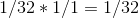Adding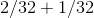gives you, as a result.

The next step is to add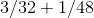. Bothandcan be multiplied by a number to produceand the smallest common shared factor is,  therefore it is the lowest common denominator.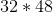would also work, but would make the math way more complicated.can be multiplied byto produce, therefore you would multiply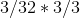, which gives you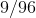can be multiplied byto produce, therefore you would multiply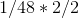, which gives you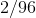.

Adding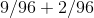gives you the final answer of.

1 2 7 8 9 10 11 12 13 15 Next →

### All Algebra II Resources